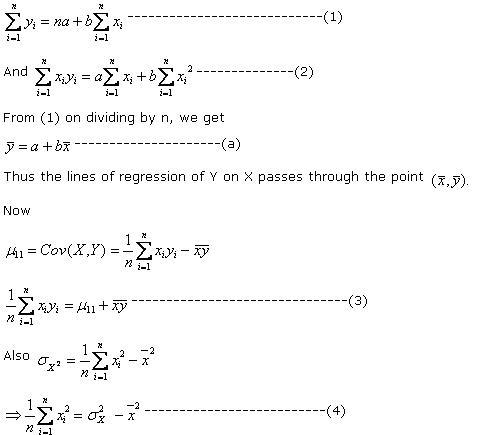# Statistics Assignment Help With Lines Of Regression

If the variables in a bivariate distribution are related, we will find the points in the scatter diagram will cluster round some curve called the “curve of regression”. If the curve is a straight line, it is called the line of regression and there is said to be linear regression between the variables, otherwise regression is said to be curvilinear.The line of regression is the line which gives the best estimate to the value of one variable for any specific value of the other variable. Thus the line of regression is the line of “best fit” and is obtained by the principle of least squares.

Let us suppose that in the bivariate distribution (xi, yi); i=1,2,……..n; Y is the dependent variable and X is independent variable. Let the line of regression of Y on X be Y = a+bX.

According to the principle of least squares, the normal equations for estimating a and b are

###Dividing (2)by n and using (3) and (4) we get